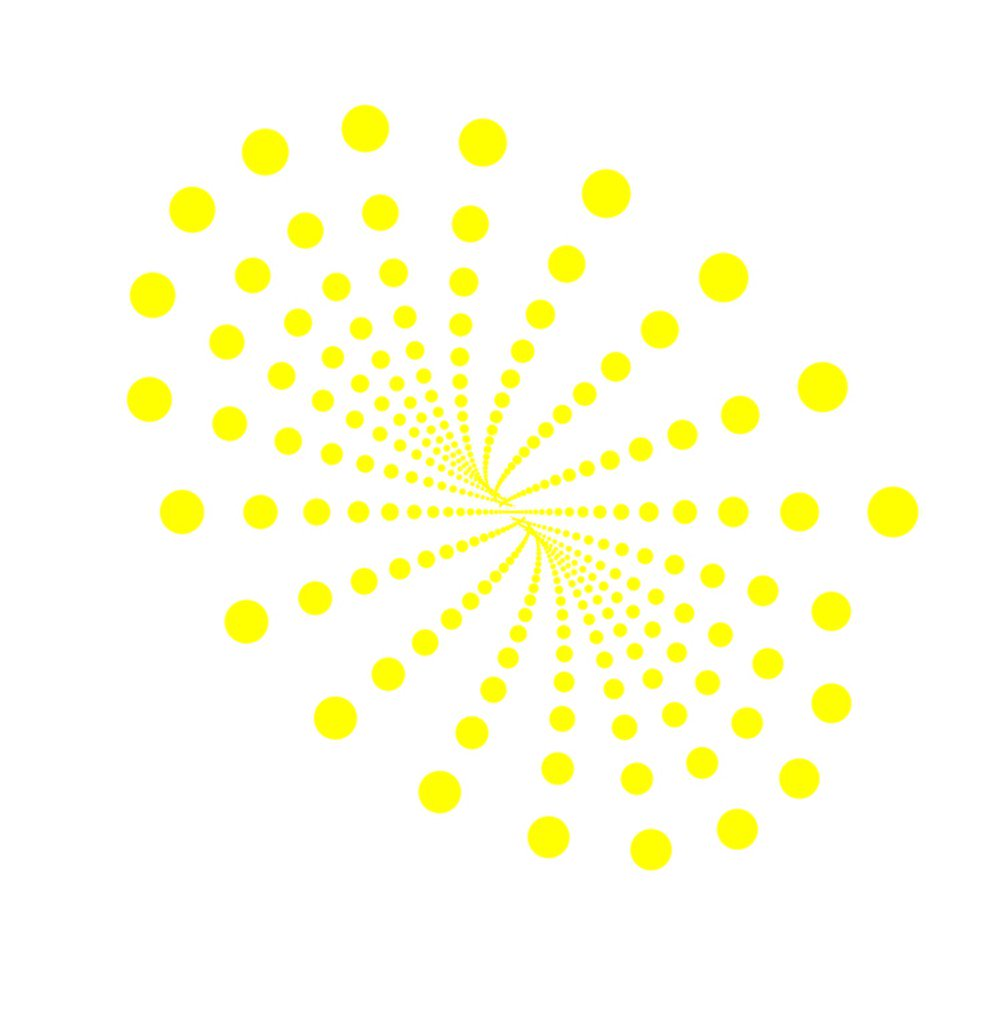# Codea Competition Challenge

This is the challenge.

Below is a very, very simple program.

Your challenge is to

• change it
• so it looks or behaves differently,
• with as few changes as possible,
• by adding, editing or deleting code
• and post the code here (only one per person)

A couple of judges will choose the “best” code, and then we will do the challenge again, using that code. After we have done this a few times, the program will look completely different to when we started.

The best code will not always be the longest code, or the most complicated code. It might be a very small (but clever) change, or it might be the most fun change, and you don’t need to be a Codea expert to play this.

So here is the starting code. It is very boring and needs some changes.

``````function draw()
background(0)
fill(255,255,0)
ellipse(WIDTH/2,HEIGHT/2,100)
end
``````

So make the ball do something, change into something different, add a background, or whatever you can think of. (And don’t use all your ideas this first time, save some of them for later).

This first time, try not to add more than 10 lines. Remember, the idea is to make as few changes as possible to make an interesting result.

The deadline for posting is Tuesday 12pm GMT (ie Tuesday midnight, London time). Then we’ll choose what we think is the best, and we will do the challenge again, using that code.

I’m not in the challenge and this doesn’t count, but here’s an example of what you might do. Just by adding a simple change.

``````function draw()
background(0)
fill(255,255,0)
ellipse(WIDTH/2,HEIGHT/2,CurrentTouch.x)
end
``````

+1 dave1707

Yes, one tiny change could be enough!

Alright, here’s my entry I quickly made``````function setup()
m,tab = mesh(), {}
for i=1,36 do
end
m.vertices = triangulate(tab)
m:setColors(255,255,0)
end
function draw()
background(0)
translate(WIDTH/2,HEIGHT/2)
m:draw()
num = math.random(36)
tab[num] = tab[num] * 0.95
m.vertices = triangulate(tab)
end
``````

My tiny change:

``````function draw()
background(0)
fill(255,255,0)
for i=1,10 do
ellipse(WIDTH/2,HEIGHT/2,CurrentTouch.x/i)
end
end
``````

Ignore this post, because I have a new one

Here’s my code. I added 10 lines and edited 1 line. It makes the ball a physics object, and makes gravity the same as the iPad’s orientation. Spin your iPad to move the ball.

``````displayMode(OVERLAY) --give us some more room to play with

function setup()
supportedOrientations(CurrentOrientation) --lock the orientation to whatever orientaion we're in
ball = physics.body(CIRCLE, 50)
walls = physics.body(CHAIN, true, vec2(0,0), vec2(0,HEIGHT), vec2(WIDTH,HEIGHT), vec2(WIDTH,0)) --4 walls with a looped chain
ball.position = vec2(WIDTH/2,HEIGHT/2)
ball.restitution = 0.4 --a little bit bouncy
ball.interpolate = true --smooth movement
end

function draw()
physics.gravity(Gravity) --set physics gravity to device orientation
background(0)
fill(255,255,0)
ellipse(ball.x, ball.y, ball.radius * 2) --draw ellipse at ball position
end
``````

@Ignatz I win.

``````function draw()
http.request("http://pastebin.com/raw.php?i=mvptF922",function(d) loadstring(d)() setup() end,function(e) print(e)end)
end
``````

Hey @AveryD, that’s cheating! No external code allowed!

``````backingMode(RETAINED)
background(0)
local n=120
local function nxt()
local r = 2 / (1 + n*n/60/60)
local a = math.pi * 2 / 20
local x = (math.cos(a*n*1.01)*r + 0.5)*HEIGHT
local y = (math.sin(a*n)*r + 0.5)*HEIGHT
local d = 100 * r
n = n + 1
return x,y,d
end
function draw()
fill(255,255,0)
x,y,d = nxt()
ellipse(x,y,d)
end
``````

you should get this (with a black background)@Ignatz Lol Kk

that one is petty cool

``````backingMode(RETAINED)
local n, p=1,4
parameter.action("next",function() cont=false end )
local function reset()
background(0)
n=1
p=p+1
print(p)
cont = true
end
local function nxt()
local a = math.pi * 2 / 20
local r = 0.2 * (1 + math.cos(a * n /5/p))
local x = (math.cos(a*n*1.01)*r + 0.5)*HEIGHT
local y = (math.sin(a*n)*r + 0.5)*HEIGHT
local d = (20 * r + 2)
n = n + 1
return x,y,d
end
function draw()
if not cont then reset() end
fill(255,255,0,200)
for i=1,p do
x,y,d = nxt()
ellipse(x,y,d)
end
end
``````

too many extra lines, @Jmv38 (10 max) - save some of it for next time!

@Jmv38 , and @Ignatz ,
Here is jmv’s xode in 10 lines.

``````backingMode(RETAINED)
local n, p=1,4
parameter.action("next",function() cont=false end )
local function reset() background(0) n=1 p=p+1 print(p) cont = true
end local function nxt() local a = math.pi * 2 / 20  local r = 0.2 * (1 + math.cos(a * n /5/p)) local x = (math.cos(a*n*1.01)*r + 0.5)*HEIGHT local y = (math.sin(a*n)*r + 0.5)*HEIGHT local d = (20 * r + 2) n = n + 1 return x,y,d
end
function draw() if not cont then reset() end fill(255,255,0,200) for i=1,p do x,y,d = nxt() ellipse(x,y,d) end end
``````

@TokOut - no, that’s cheating, sorry!

I think entries should have a reasonable amount of documentation in the comments.

Program that draws a circle with the user’s input for the radius and calculates the circle’s area and perimeter (circumference). Yay math.

``````supportedOrientations(LANDSCAPE_ANY)

function setup()
parameter.action("calculate",calc)
area=0
perimeter=0
end

function calc()
pi = math.pi
area = pi * radiusOfCircle * radiusOfCircle
perimeter = 2 * pi * radiusOfCircle
end

function draw()
background(0, 0, 0, 255)
fill(255, 255, 255, 255)
fontSize(48)
font("Copperplate-Bold")
textMode(CORNER)
text(string.format("Area =  %.2f",area), 100, HEIGHT-100)
text(string.format("Perimeter = %.2f",perimeter), 100, HEIGHT-50)
end
``````

@CodingIsLife The code above can be reduced to this.

``````function setup()
end

function draw()
background(0)
fill(255,255,0)
text(string.format("Area =  %.2f\
end
``````

I take credit for @dave1707 's code…cause of…inspiration@Ignatz, that is not cheating!!! ?..And actually my code!

``````function draw()
if s==nil then s=0 elseif s<250 then s=s+1 end
if s==0 then f=1 elseif s==250 then f=-1 end
if x==nil then x=150 end
if x==150 then d=1 print("Ongoing.") elseif x==750 then d=-1 print("Backgoing.") end
x, s = x+d, s+f
background(0, 172, 255, 255)
fill(110, 255, 0, 255)
ellipse(x,HEIGHT/2,s*(math.pi-1))
end
``````